Olympiad Test : Analogy - 5

# Olympiad Test : Analogy - 5

Test Description

## 10 Questions MCQ Test Maths Olympiad Class 6 | Olympiad Test : Analogy - 5

Olympiad Test : Analogy - 5 for Class 6 2022 is part of Maths Olympiad Class 6 preparation. The Olympiad Test : Analogy - 5 questions and answers have been prepared according to the Class 6 exam syllabus.The Olympiad Test : Analogy - 5 MCQs are made for Class 6 2022 Exam. Find important definitions, questions, notes, meanings, examples, exercises, MCQs and online tests for Olympiad Test : Analogy - 5 below.
Solutions of Olympiad Test : Analogy - 5 questions in English are available as part of our Maths Olympiad Class 6 for Class 6 & Olympiad Test : Analogy - 5 solutions in Hindi for Maths Olympiad Class 6 course. Download more important topics, notes, lectures and mock test series for Class 6 Exam by signing up for free. Attempt Olympiad Test : Analogy - 5 | 10 questions in 20 minutes | Mock test for Class 6 preparation | Free important questions MCQ to study Maths Olympiad Class 6 for Class 6 Exam | Download free PDF with solutions
 1 Crore+ students have signed up on EduRev. Have you?
Olympiad Test : Analogy - 5 - Question 1

### The second figure in the first unit of problem figures bears a certain relationship to the first figure. Similarly, one of the figures in the answer figures bears the same relationship to the first figure in the second unit of the problem figures. You are therefore to locate the figure which would fit the question mark. Problem Figures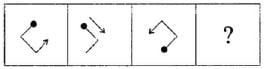Answer Figures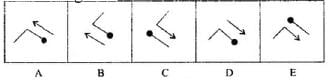Detailed Solution for Olympiad Test : Analogy - 5 - Question 1

Black dot should be in right corner with opposite direction of arrow and arrow should be under the black dot.

Olympiad Test : Analogy - 5 - Question 2

### The second figure in the first unit of problem figures bears a certain relationship to the first figure. Similarly, one of the figures in the answer figures bears the same relationship to the first figure in the second unit of the problem figures. You are therefore to locate the figure which would fit the question mark. Problem Figures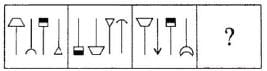Answer Figures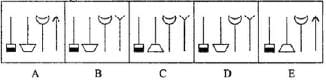Detailed Solution for Olympiad Test : Analogy - 5 - Question 2

Third image should be in first place and gets inverted. First image should be in second place and gets inverted. Second image should be in fourth place and only outer part be inverted. We have to follow same pattern with fourth image.

Olympiad Test : Analogy - 5 - Question 3

### The second figure in the first unit of problem figures bears a certain relationship to the first figure. Similarly, one of the figures in the answer figures bears the same relationship to the first figure in the second unit of the problem figures. You are therefore to locate the figure which would fit the question mark. Problem Figures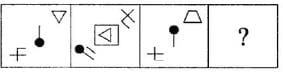Answer Figures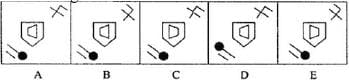Detailed Solution for Olympiad Test : Analogy - 5 - Question 3

As per pattern trapezium should be in middle inside the pentagon with ninety degree rotation. Similar patter

Olympiad Test : Analogy - 5 - Question 4

In the following question, four words have been given out of which three are alike in some maner, while the fourth one is different. Choose the word which is different from the rest.

Detailed Solution for Olympiad Test : Analogy - 5 - Question 4

The mayor, governor, and senator are all persons elected to government offices; the lawyer is not an elected official.

Olympiad Test : Analogy - 5 - Question 5

The second figure in the first unit of problem figures bears a certain relationship to the first figure. Similarly, one of the figures in the answer figures bears the same relationship to the first figure in the second unit of the problem figures. You are therefore to locate the figure which would fit the question mark.
Problem Figures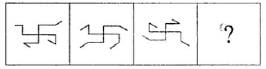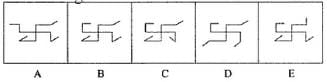Detailed Solution for Olympiad Test : Analogy - 5 - Question 5

All the outer parts gets inside and inner part gets outside.

Olympiad Test : Analogy - 5 - Question 6

The second figure in the first unit of problem figures bears a certain relationship to the first figure. Similarly, one of the figures in the answer figures bears the same relationship to the first figure in the second unit of the problem figures. You are therefore to locate the figure which would fit the question mark.
Problem Figures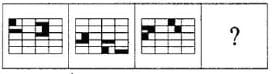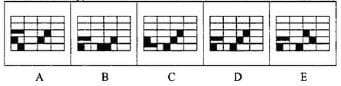Detailed Solution for Olympiad Test : Analogy - 5 - Question 6

Shifting the shaded portion in opposite direction, we get the required image.

Olympiad Test : Analogy - 5 - Question 7

The second figure in the first unit of problem figures bears a certain relationship to the first figure. Similarly, one of the figures in the answer figures bears the same relationship to the first figure in the second unit of the problem figures. You are therefore to locate the figure which would fit the question mark.
Problem Figures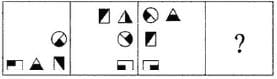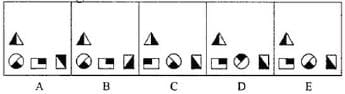Detailed Solution for Olympiad Test : Analogy - 5 - Question 7

All the figures get inverted.

Olympiad Test : Analogy - 5 - Question 8

The second figure in the first unit of problem figures bears a certain relationship to the first figure. Similarly, one of the figures in the answer figures bears the same relationship to the first figure in the second unit of the problem figures. You are therefore to locate the figure which would fit the question mark.
Problem Figures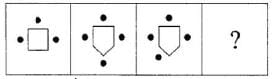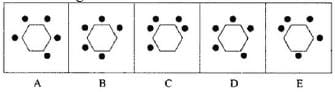Detailed Solution for Olympiad Test : Analogy - 5 - Question 8

The number of dots is one less than the number of sides.

Olympiad Test : Analogy - 5 - Question 9

The second figure in the first unit of problem figures bears a certain relationship to the first figure. Similarly, one of the figures in the answer figures bears the same relationship to the first figure in the second unit of the problem figures. You are therefore to locate the figure which would fit the question mark.
Problem Figures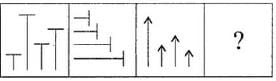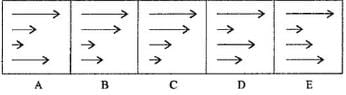Detailed Solution for Olympiad Test : Analogy - 5 - Question 9

The number of positions of arrow are changed in order.

Olympiad Test : Analogy - 5 - Question 10

The second figure in the first unit of problem figures bears a certain relationship to the first figure. Similarly, one of the figures in the answer figures bears the same relationship to the first figure in the second unit of the problem figures. You are therefore to locate the figure which would fit the question mark.
Problem Figures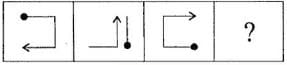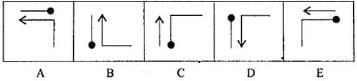Detailed Solution for Olympiad Test : Analogy - 5 - Question 10

As per pattern the image includes arrow rotated through 90° and then inverts. The image with black dot should be in opposite direction with arrow.

## Maths Olympiad Class 6

43 tests
 Use Code STAYHOME200 and get INR 200 additional OFF Use Coupon Code
Information about Olympiad Test : Analogy - 5 Page
In this test you can find the Exam questions for Olympiad Test : Analogy - 5 solved & explained in the simplest way possible. Besides giving Questions and answers for Olympiad Test : Analogy - 5, EduRev gives you an ample number of Online tests for practice

43 tests